# Convergence/Divergence Of Series Notes | Study Algebra for IIT JAM Mathematics - Mathematics

## Mathematics: Convergence/Divergence Of Series Notes | Study Algebra for IIT JAM Mathematics - Mathematics

The document Convergence/Divergence Of Series Notes | Study Algebra for IIT JAM Mathematics - Mathematics is a part of the Mathematics Course Algebra for IIT JAM Mathematics.
All you need of Mathematics at this link: Mathematics

In the previous section we spent some time getting familiar with series and we briefly defined convergence and divergence. Before worrying about convergence and divergence of a series we wanted to make sure that we’ve started to get comfortable with the notation involved in series and some of the various manipulations of series that we will, on occasion, need to be able to do.
As noted in the previous section most of what we were doing there won’t be done much in this chapter. So, it is now time to start talking about the convergence and divergence of a series as this will be a topic that we’ll be dealing with to one extent or another in almost all of the remaining sections of this chapter.
So, let’s recap just what an infinite series is and what it means for a series to be convergent or divergent. We’ll start with a sequence {an}n=1 and again note that we’re starting the sequence at n=1 only for the sake of convenience and it can, in fact, be anything.
Next, we define the partial sums of the series as,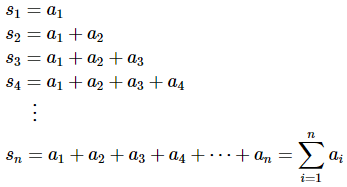and these form a new sequence, {sn}n=1.
An infinite series, or just series here since almost every series that we’ll be looking at will be an infinite series, is then the limit of the partial sums. Or,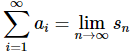It is important to remember that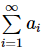is really nothing more than a convenient notation for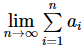so we do not need to keep writing the limit down. We do, however, always need to remind ourselves that we really do have a limit there!
If the sequence of partial sums is a convergent sequence (i.e. its limit exists and is finite) then the series is also called convergent and in this case if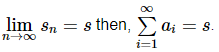Likewise, if the sequence of partial sums is a divergent sequence (i.e. its limit doesn’t exist or is plus or minus infinity) then the series is also called divergent.
Let’s take a look at some series and see if we can determine if they are convergent or divergent and see if we can determine the value of any convergent series we find.

Example 1: Determine if the following series is convergent or divergent. If it converges determine its value.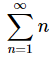Solution. To determine if the series is convergent we first need to get our hands on a formula for the general term in the sequence of partial sums.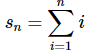This is a known series and its value can be shown to be,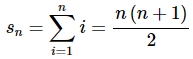Don’t worry if you didn’t know this formula (we’d be surprised if anyone knew it…) as you won’t be required to know it in my course.
So, to determine if the series is convergent we will first need to see if the sequence of partial sums,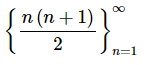is convergent or divergent. That’s not terribly difficult in this case. The limit of the sequence terms is,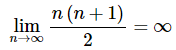Therefore, the sequence of partial sums diverges to ∞ and so the series also diverges.
So, as we saw in this example we had to know a fairly obscure formula in order to determine the convergence of this series. In general finding a formula for the general term in the sequence of partial sums is a very difficult process. In fact after the next section we’ll not be doing much with the partial sums of series due to the extreme difficulty faced in finding the general formula. This also means that we’ll not be doing much work with the value of series since in order to get the value we’ll also need to know the general formula for the partial sums.
We will continue with a few more examples however, since this is technically how we determine convergence and the value of a series. Also, the remaining examples we’ll be looking at in this section will lead us to a very important fact about the convergence of series.
So, let’s take a look at a couple more examples.

Example 2: Determine if the following series converges or diverges. If it converges determine its sum.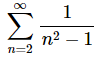Solution. This is actually one of the few series in which we are able to determine a formula for the general term in the sequence of partial fractions. However, in this section we are more interested in the general idea of convergence and divergence and so we’ll put off discussing the process for finding the formula until the next section.
The general formula for the partial sums is,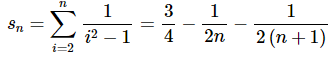and in this case we have,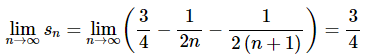The sequence of partial sums converges and so the series converges also and its value is,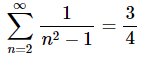Example 3: Determine if the following series converges or diverges. If it converges determine its sum.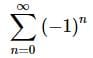Solution. In this case we really don’t need a general formula for the partial sums to determine the convergence of this series. Let’s just write down the first few partial sums.
s0=1
s1=1−1=0
s2=1−1+1=1
s3=1−1+1−1=0
etc.
So, it looks like the sequence of partial sums is,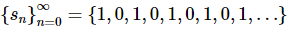and this sequence diverges since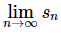doesn’t exist. Therefore, the series also diverges.

Example 4: Determine if the following series converges or diverges. If it converges determine its sum.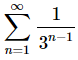Solution. Here is the general formula for the partial sums for this series.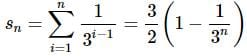Again, do not worry about knowing this formula. This is not something that you’ll ever be asked to know in my class.
In this case the limit of the sequence of partial sums is,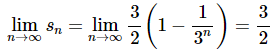The sequence of partial sums is convergent and so the series will also be convergent. The value of the series is,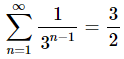As we already noted, do not get excited about determining the general formula for the sequence of partial sums. There is only going to be one type of series where you will need to determine this formula and the process in that case isn’t too bad. In fact, you already know how to do most of the work in the process as you’ll see in the next section.
So, we’ve determined the convergence of four series now. Two of the series converged and two diverged. Let’s go back and examine the series terms for each of these. For each of the series let’s take the limit as n goes to infinity of the series terms (not the partial sums!!)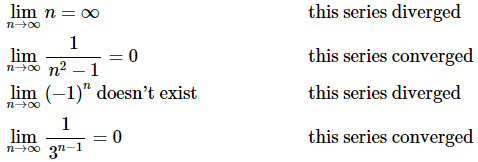Notice that for the two series that converged the series term itself was zero in the limit. This will always be true for convergent series and leads to the following theorem.

Theorem
If ∑an  converges then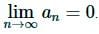Proof
First let’s suppose that the series starts at n=1. If it doesn’t then we can modify things as appropriate below. Then the partial sums are,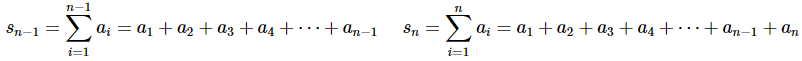Next, we can use these two partial sums to write,
an=sn−sn−1
Now because we know that ∑ais convergent we also know that the sequence {sn}n=1 is also convergent and that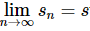for some finite value s. However, since n−1→∞ as n→∞ we also have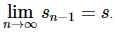We now have,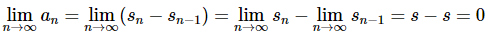Be careful to not misuse this theorem! This theorem gives us a requirement for convergence but not a guarantee of convergence. In other words, the converse is NOT true. If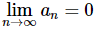the series may actually diverge! Consider the following two series.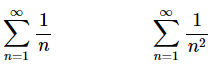In both cases the series terms are zero in the limit as n goes to infinity, yet only the second series converges. The first series diverges. It will be a couple of sections before we can prove this, so at this point please believe this and know that you’ll be able to prove the convergence of these two series in a couple of sections.
Again, as noted above, all this theorem does is give us a requirement for a series to converge. In order for a series to converge the series terms must go to zero in the limit. If the series terms do not go to zero in the limit then there is no way the series can converge since this would violate the theorem.
This leads us to the first of many tests for the convergence/divergence of a series that we’ll be seeing in this chapter.

Divergence Test
If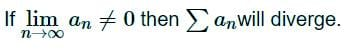Again, do NOT misuse this test. This test only says that a series is guaranteed to diverge if the series terms don’t go to zero in the limit. If the series terms do happen to go to zero the series may or may not converge! Again, recall the following two series,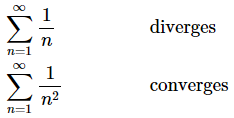One of the more common mistakes that students make when they first get into series is to assume that if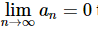then ∑an will converge. There is just no way to guarantee this so be careful!
Let’s take a quick look at an example of how this test can be used.

Example 5: Determine if the following series is convergent or divergent.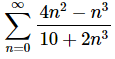Solution. With almost every series we’ll be looking at in this chapter the first thing that we should do is take a look at the series terms and see if they go to zero or not. If it’s clear that the terms don’t go to zero use the Divergence Test and be done with the problem.
That’s what we’ll do here.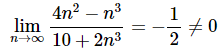The limit of the series terms isn’t zero and so by the Divergence Test the series diverges.
The divergence test is the first test of many tests that we will be looking at over the course of the next several sections. You will need to keep track of all these tests, the conditions under which they can be used and their conclusions all in one place so you can quickly refer back to them as you need to.
Next we should briefly revisit arithmetic of series and convergence/divergence. As we saw in the previous section if ∑an and ∑bn are both convergent series then so are ∑can and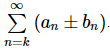Furthermore, these series will have the following sums or values.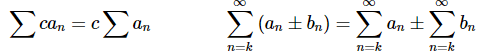We’ll see an example of this in the next section after we get a few more examples under our belt. At this point just remember that a sum of convergent series is convergent and multiplying a convergent series by a number will not change its convergence.
We need to be a little careful with these facts when it comes to divergent series. In the first case if ∑an is divergent then ∑can will also be divergent (provided c isn’t zero of course) since multiplying a series that is infinite in value or doesn’t have a value by a finite value (i.e. c) won’t change the fact that the series has an infinite or no value. However, it is possible to have both ∑an and ∑bn be divergent series and yet have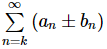be a convergent series.
Now, since the main topic of this section is the convergence of a series we should mention a stronger type of convergence. A series ∑an is said to converge absolutely if ∑|an| also converges. Absolute convergence is stronger than convergence in the sense that a series that is absolutely convergent will also be convergent, but a series that is convergent may or may not be absolutely convergent.
In fact if ∑an converges and ∑|an| diverges the series ∑an is called conditionally convergent.
At this point we don’t really have the tools at hand to properly investigate this topic in detail nor do we have the tools in hand to determine if a series is absolutely convergent or not. So we’ll not say anything more about this subject for a while. When we finally have the tools in hand to discuss this topic in more detail we will revisit it. Until then don’t worry about it. The idea is mentioned here only because we were already discussing convergence in this section and it ties into the last topic that we want to discuss in this section.
In the previous section after we’d introduced the idea of an infinite series we commented on the fact that we shouldn’t think of an infinite series as an infinite sum despite the fact that the notation we use for infinite series seems to imply that it is an infinite sum. It’s now time to briefly discuss this.
First, we need to introduce the idea of a rearrangement. A rearrangement of a series is exactly what it might sound like, it is the same series with the terms rearranged into a different order.
For example, consider the following infinite series.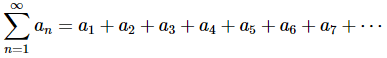A rearrangement of this series is,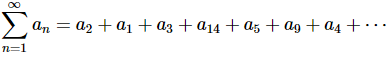The issue we need to discuss here is that for some series each of these arrangements of terms can have different values despite the fact that they are using exactly the same terms.
Here is an example of this. It can be shown that,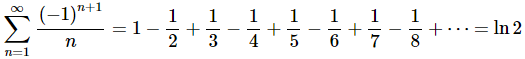(1.)
Since this series converges we know that if we multiply it by a constant c its value will also be multiplied by c. So, let’s multiply this by 1/2 to get,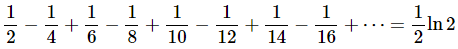(2.)
Now, let’s add in a zero between each term as follows.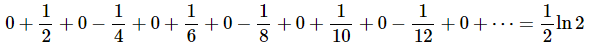(3.)
Note that this won’t change the value of the series because the partial sums for this series will be the partial sums for the (2) except that each term will be repeated. Repeating terms in a series will not affect its limit however and so both (2) and (3) will be the same.
We know that if two series converge we can add them by adding term by term and so add (1) and (3) to get,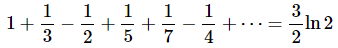Now, notice that the terms of (4) are simply the terms of (1) rearranged so that each negative term comes after two positive terms. The values however are definitely different despite the fact that the terms are the same.
Note as well that this is not one of those “tricks” that you see occasionally where you get a contradictory result because of a hard to spot math/logic error. This is a very real result and we’ve not made any logic mistakes/errors.
Here is a nice set of facts that govern this idea of when a rearrangement will lead to a different value of a series.
Facts
Given the series∑an,
1. ∑an is absolutely convergent and its value is s then any rearrangement of ∑an will also have a value of s.
2. If ∑an is conditionally convergent and r is any real number then there is a rearrangement of ∑an whose value will be r.
Again, we do not have the tools in hand yet to determine if a series is absolutely convergent and so don’t worry about this at this point. This is here just to make sure that you understand that we have to be very careful in thinking of an infinite series as an infinite sum. There are times when we can (i.e. the series is absolutely convergent) and there are times when we can’t (i.e. the series is conditionally convergent).
As a final note, the fact above tells us that the series,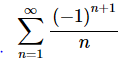must be conditionally convergent since two rearrangements gave two separate values of this series. Eventually it will be very simple to show that this series is conditionally convergent.

The document Convergence/Divergence Of Series Notes | Study Algebra for IIT JAM Mathematics - Mathematics is a part of the Mathematics Course Algebra for IIT JAM Mathematics.
All you need of Mathematics at this link: MathematicsUse Code STAYHOME200 and get INR 200 additional OFF

## Algebra for IIT JAM Mathematics

182 videos|58 docs

Track your progress, build streaks, highlight & save important lessons and more!

,

,

,

,

,

,

,

,

,

,

,

,

,

,

,

,

,

,

,

,

,

;